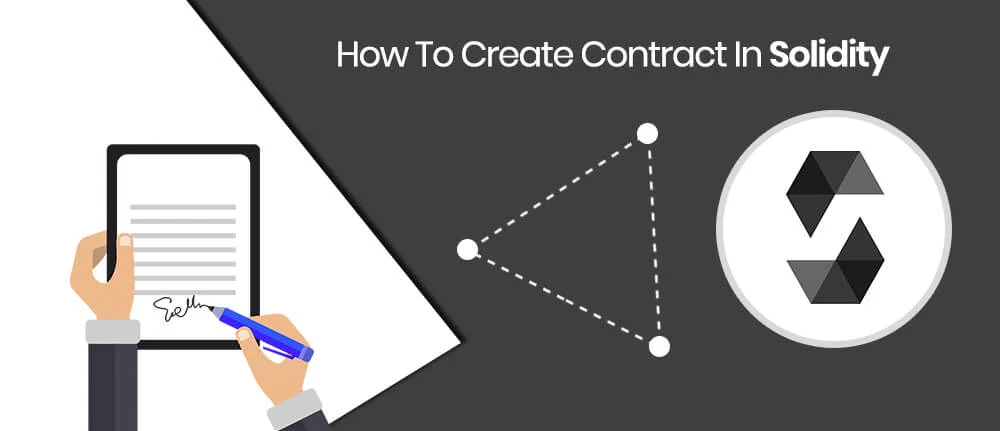﻿ How to create contract in solidity

# How to create contract in solidity

## Straighten your back and cherish with coffee - PLAY !## What is solidity

Solidity is a high-level, contract-oriented programming language for writing and implementing smart contracts in Blockchain. Blockchain development companies in USA use Solidity, Ethereum and nodejs extensively for Blockchain projects. It was influenced by C++, Python and JavaScript, Solidity is originally designed to target the Ethereum Virtual Machine (EVM).

## What is smart contract

Smart contracts are software programs that run on a blockchain. Smart contract is responsible for transferring digital currency or token between users in blockchain development.

Solidity contract code starts with contract Keyword. And its extension is .sol

Here we develop one mathematical

### solidity smart contract

for example, that performs basic Maths operation like Addition, Substitution, Multiplication, Division to illustrate how Solidity is used for writing Smart contracts.
```contract Mathematics {

}
```

lets save this in file name Mathematics.sol

## What is Solidity call function

Solidity call functions is a read-only local invocation of a contract function that does not broadcast or send anything on the blockchain.

Function does not consume any Ether to make call on solidity contract, it is identified using constant keyword.

In our example contract, we add four solidity read-only functions for all four Maths operations.

## Read More: Basics Of Solidity, Parity, Json-rpc And Visual Studio Code

```function getSum(uint a, uint b) constant returns (uint) {
return a + b;
}
```

Here a and b are given parameters for function and it returns sum. Same as for other operations we add four functions namely getSub, getMultiplication, and getDivision. One can review the whole contact code as follows.

## What is Solidity transactions

Transaction is used to send or write data to blockchain. It uses gas and Ether to send data on blockchain.

In our example contract, we find simple interest using solidity smart contract. It is a transaction function. We can not use constant keyword as function in solidity. So contract function is coded like this.

```function setInterest(uint a) {
rate = a;
}
```

In transaction we can return value but currently we have not used it in our example.

## What is events in smart contract

Solidity Events are inheritable members of contracts and that allow the convenient usage of the EVM logging facilities, this is used to “call” JavaScript callbacks functions in the user interface of a dapp, which listen for these events.

Solidity Events are defined with event keyword in smart contract. For example: I want to create events for Mathematics contract for addition result then it’s syntax looks like this

```event eventName(Typeofvariable variablename);
```

Here event is keyword identifier and sum is event name. We add event in our setInterest function so it now look like this.

```function setInterest(uint a) {
rate = a;
InterestSet(rate);
}
```

## What is modifier in smart contract

Solidity Modifier is used to automatically check conditions or change the behavior before executing smart contract. So custom software development company uses modifier to increase performance of

#### blockchain contracts

. Modifier is also inheritable properties of smart contracts and overridden by parent smart contracts.

For an example, in Division operation number can not be divided by 0. So in our contract, we check that second number is grater than or not equal to 0. then syntax looks like this.

```modifier name(){  …. }
```

In our example, notzero checks modifier for division operation is given below.

```modifier notZero(uint a){
if(a == 0){
throw;
}
_;
}
```

Here _  represent continue with next execution. We add this modifier to our solidity getDivision function so our function will check notzero condition before giving result.

## Enquire Today

```function getDivision(uint a, uint b) constant notZero(b) returns (uint) {
return a / b;
}
```

So our final Solidity Smart Contract looks like this.

```pragma solidity ^0.4.15;

contract Mathematics {

uint rate;
event InterestSet(uint a);

modifier notZero(uint a){
if(a == 0){
throw;
}
_;
}

function getSum(uint a, uint b) constant returns (uint) {
return a + b;
}

function getSub(uint a, uint b) constant returns (uint) {
return a - b;
}

function getMultiplication(uint a, uint b) constant returns (uint) {
return a * b;
}

function getDivision(uint a, uint b) constant notZero(b) returns (uint) {
return a / b;
}

function setInterest(uint a) notZero(a){
rate = a;
InterestSet(rate);
}

function getSimpleInterest(uint amount, uint month) constant notZero(amount) notZero(month) returns(uint){
return  (amount * month * rate)/100;
}

}
```

Now we can deploy this contract in parity or using truffle to check how it works. This is very basic contract to get started with solidity. We will show you this in our next blog how to deploy solidity contracts in parity and truffle. Until you can check more contacts example at solidity-by-example.